# Semiconductors - JEE Main Previous Year Questions with Solutions

JEE Main Previous Year Question of Physics with Solutions are available here. Practicing JEE Main Previous Year Papers Questions of Physics will help all the JEE aspirants in realizing the question pattern as well as help in analyzing their weak & strong areas. Get detailed Class 11th &12th Physics Notes to prepare for Boards as well as competitive exams like IIT JEE, NEET etc. eSaral helps the students in clearing and understanding each topic in a better way. eSaral is providing complete chapter-wise notes of Class 11th and 12th both for all subjects. Besides this, eSaral also offers NCERT Solutions, Previous year questions for JEE Main and Advance, Practice questions, Test Series for JEE Main, JEE Advanced and NEET, Important questions of Physics, Chemistry, Math, and Biology and many more. Download eSaral app for free study material and video tutorials.   Simulator Previous Years AIEEE/JEE Mains Questions
Q. An p-n junction (D) shown in the figure can act as a rectifier. An alternating current source (V) is connected in the circuit. The current (I) in the resistor R can be shown by: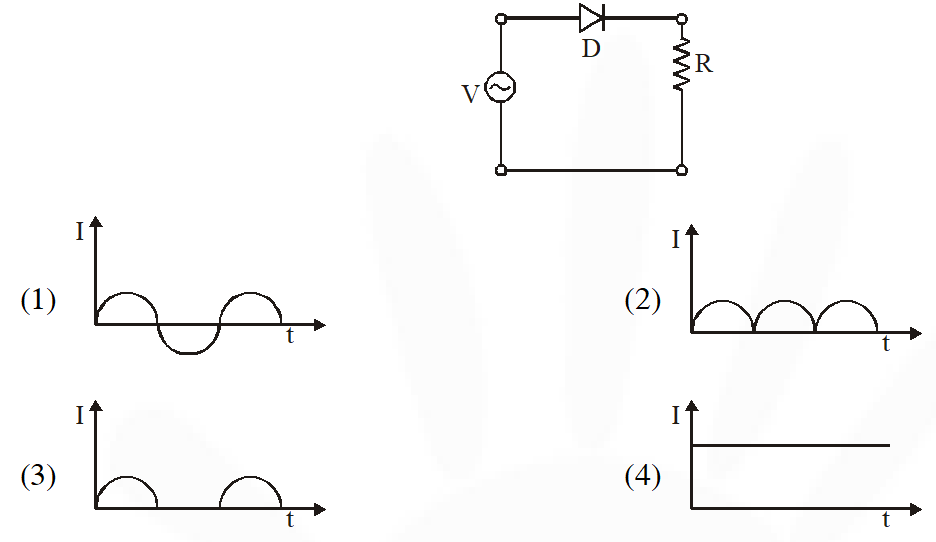[AIEEE-2009]
Ans. (3) When postive cycle enters into diode it is forward bias while for negative half cycle it is reversed bias.
Q. The logic circuit shown below has the input wave forms ‘A’ and ‘B’ as shown. Pick out the correct output waveform.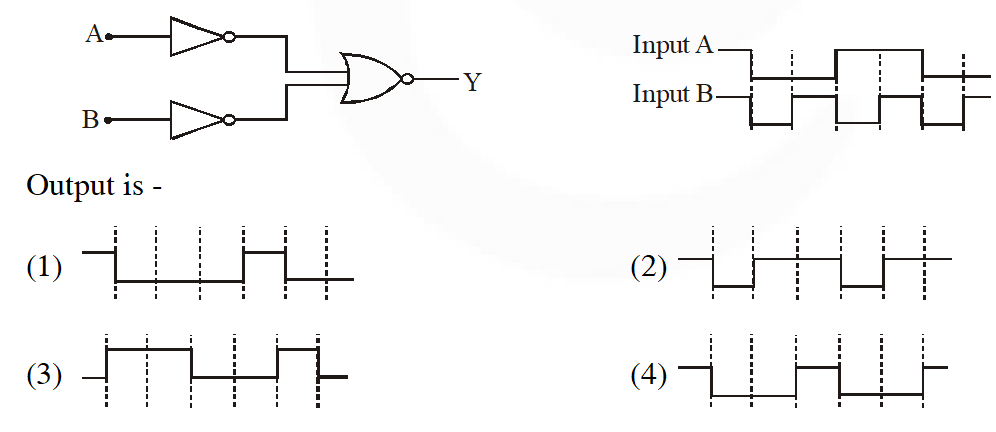[AIEEE-2009]
Ans. (1)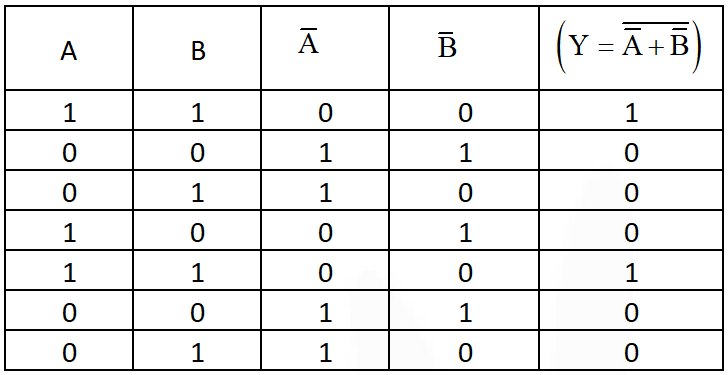Q. The combination of gates shown below yields.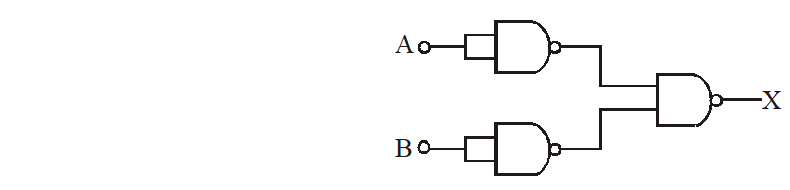(1) XOR gate (2) NAND gate (3) OR gate (4) NOT gate [AIEEE-2010]
Ans. (3) No need
Q. Truth table for system of four NAND gates as shown in figure is: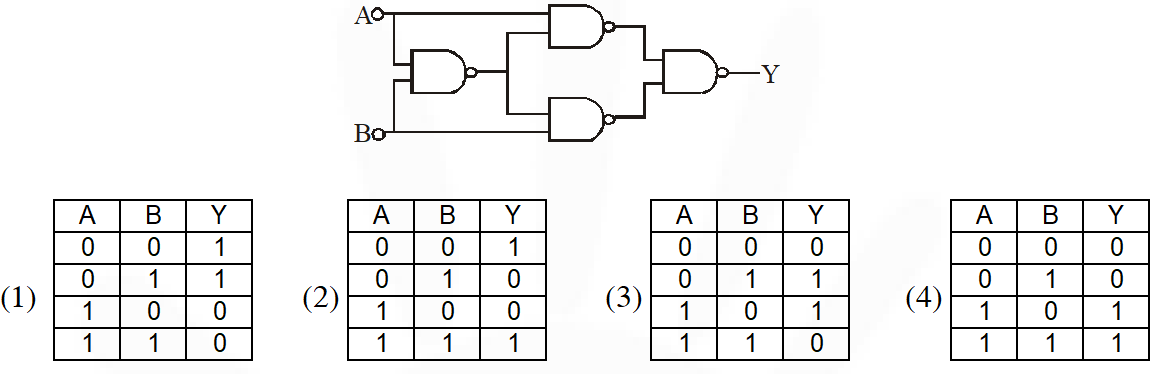[AIEEE-2012]
Ans. (3) No need
Q. The I-V characteristic of an LED is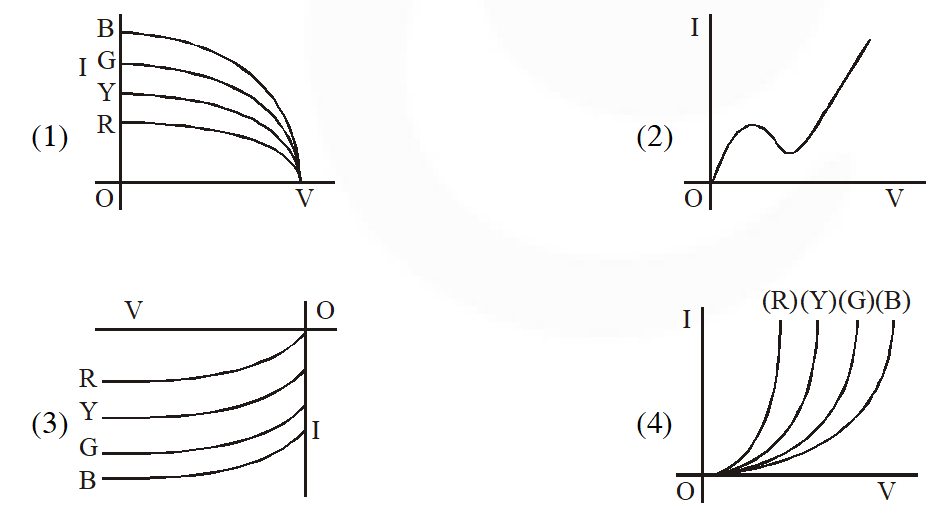[JEE Main 2013]
Ans. (4) I $\alpha \frac{1}{\lambda}$ more $\lambda,$ less intensity and less energy.
Q. The forward biased diode connection is: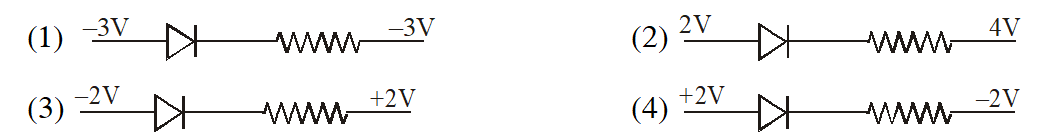[JEE Main-2014]
Ans. (4) If n side is at low potential relative to P side then diode is forward bias.
Q. For a common emitter configuration, if $\alpha$ and $\beta$ have their usual meanings, the incorrect relationship between $\alpha$ and $\beta$ is $(1) \alpha=\frac{\beta^{2}}{1+\beta^{2}}$ (2) $\frac{1}{\alpha}=\frac{1}{\beta}+1$ (3) $\alpha=\frac{\beta}{1-\beta}$ (4) $\alpha=\frac{\beta}{1+\beta}$ [JEE Main-2016]
Ans. (1,3) $\alpha=\frac{I_{C}}{I_{e}}, \beta=\frac{I_{C}}{I_{b}}$ $I_{e}=I_{b}+I_{c}$ $\Rightarrow \frac{I_{e}}{I_{c}}=\frac{I_{b}}{I_{c}}+1 \quad \Rightarrow \quad \frac{1}{\alpha}=\frac{1}{\beta}+1$
Q. If a, b, c, d are inputs to a gate and x is its output, then as per the following time graph, the gate is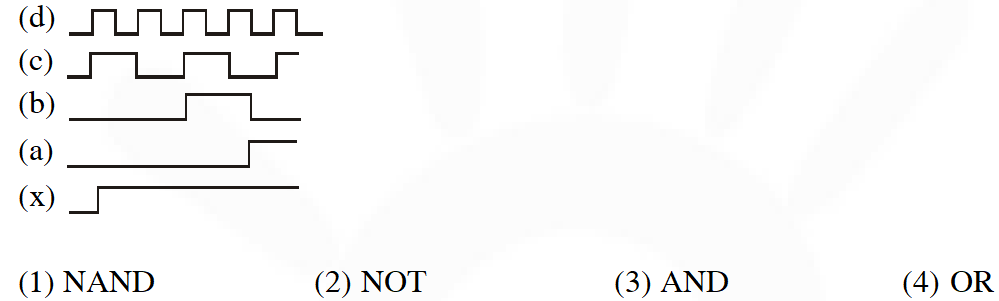[JEE Main-2016]
Ans. (4) Output of OR gate is 0 when all inputs are 0 & output is 1 when atleast one of the input is 1. Observing output x :- It is 0 when all inputs are 0 & it is 1 when atleast one of the inputs is 1. $\therefore$ OR gate
Q. The temperature dependence of resistances of Cu and undoped Si in the temperature range 300-400K, is best described by :- (1) Linear decrease for Cu, linear decrease for Si. (2) Linear increase for Cu, linear increase for Si. (3) Linear increase for Cu, exponential increase for Si (4) Linear increase for Cu, exponential decrease for Si [JEE Main-2016]
Ans. (4) Factual Cu is conductor so with increase in temperature, resistance will increase Si is semiconductor so with increase in temperature resistance will decrease
Q. Identify the semiconductor devices whose characteristics are given below, in the order (a), (b), (c), (d) :-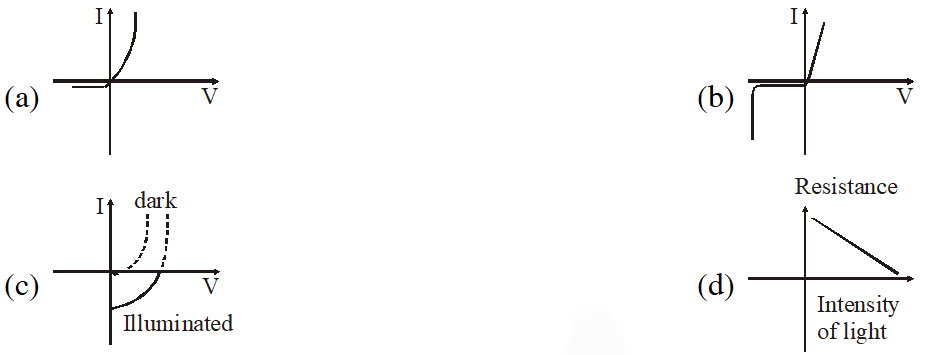(1) Zener diode, Solar cell, Simple diode, Light dependent resistance (2) Simple diode, Zener diode, Solar cell, Light dependent resistance (3) Zener diode, Simple diode, Light dependent resistance, Solar cell (4) Solar cell, Light dependent resistance, Zener diode, Simple diode [JEE Main-2016]
Ans. (2) Factual
Q. In a common emitter amplifier circuit using an n-p-n transistor, the phase difference between the input and the output voltages will be : (1) $135^{\circ}$ (2) $180^{\circ}$ (3) $45^{\circ}$ (4) $90^{\circ}$ [JEE Main-2017]
Ans. (2) In common emitter amplifier circuit input and out put voltage are out of phase. When input voltage is increased then $\mathrm{i}_{\mathrm{b}}$ is increased, i also increases so voltage drop across $\mathrm{R}_{\mathrm{c}}$ is increased. However increase in voltage across $\mathrm{R}_{\mathrm{C}}$ is in opposite sense.
Q. The reading of the ammeter for a silicon diode in the given circuit is :-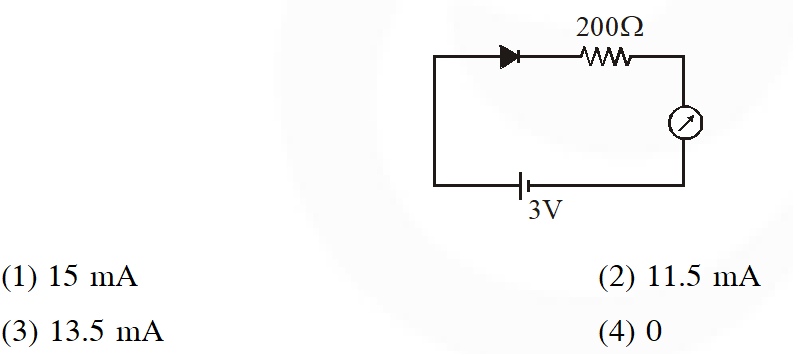[JEE Main-2018]
Ans. (2)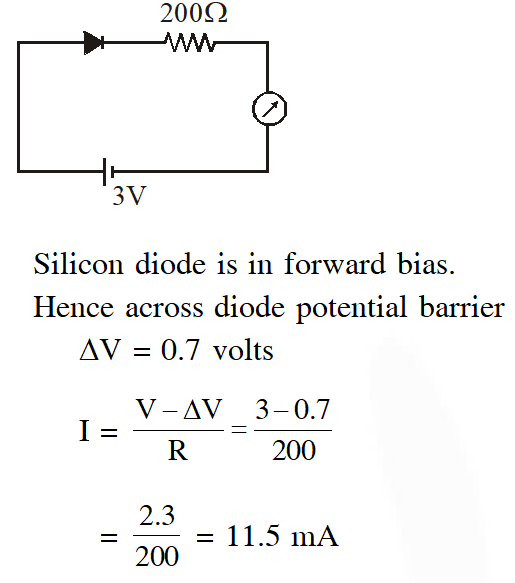anketashrivakealaman
April 1, 2022, 6:09 p.m.
thankss
Prashant
Dec. 8, 2021, 8:15 p.m.
Thanxx sir 🙏
prince
Dec. 3, 2021, 5:17 p.m.
i am acceited the alredy 80-90% correct love from qution
Patil pruthvi
Sept. 19, 2021, 8 p.m.
The question is the very good for jee main plzz solve the questions and crack for the jee main thank you eSaral
Yusuf
Aug. 11, 2021, 3:22 p.m.
Thank you so much 👍
shubha
July 26, 2021, 5:43 p.m.
thank u so much
Chappri nibba
July 21, 2021, 10:33 a.m.
Thanks from my side💋💌
Diptiranjan Sahoo
June 1, 2021, 1:07 p.m.
Thanks but we need more questions. Please upload more previous year questions
Nirupam
May 28, 2021, 7:27 a.m.
It will be better, to have solutions along with the correct answer. It is time saving as one can easily figure out their mistakes.
Aashu kumar
May 13, 2021, 11:59 a.m.
Good!
UJJWAL
April 20, 2021, 11:20 a.m.
BEST SOLUTIONS ACHHE BANAO
Neelu Sahu
April 3, 2021, 9:01 p.m.
Thanks 😊😘
karaN
March 25, 2021, 1:02 p.m.
tq
armaan
March 25, 2021, 2:46 a.m.
good collection of question
Shaheen
March 14, 2021, 7:29 a.m.
Thank you
Yashaswini
March 11, 2021, 10:03 p.m.
Once check a,b,c,d in puts ques
Tanuja
March 6, 2021, 7:47 p.m.
Ruhi
March 5, 2021, 5:04 p.m.
Thank you 😊 so much 💕 esaral
blaze
March 4, 2021, 7:25 p.m.
M. Rithvik
Feb. 23, 2021, 9:47 p.m.
It was wow Thank you 😊👍✌
M. Rithvik
Feb. 23, 2021, 9:47 p.m.
It was helpful Thank you 😊👍✌
Neha
Feb. 22, 2021, 5:51 p.m.
It was helpful.. Thank you ☺️
om
Feb. 20, 2021, 8:58 p.m.
wow
ammulu
Feb. 2, 2021, 3:19 p.m.
super
Sarayu
Jan. 22, 2021, 8:39 a.m.
Thanks hope you will make avalilabilty some more questions
Jan. 18, 2021, 12:44 p.m.
Great app sir
Shranika
Jan. 6, 2021, 10:39 p.m.
helped me a lot thq
Tabinda
Dec. 29, 2020, 5:14 p.m.
Usefull app
SNEHA
Dec. 29, 2020, 9:30 a.m.
Useful &amp; thanks 😊
Esaaraal ka baap
Dec. 25, 2020, 8:45 p.m.
Mogambo khush hua
akash bartwal
Nov. 2, 2020, 6:19 p.m.
solution of aieee 2012 is wrong the correct answer is (2)
VENAT
Sept. 4, 2020, 11:53 a.m.
Good one, Thanks
SURYA
Sept. 2, 2020, 9:40 a.m.
LATEST SOLUTIONS KAHA HE ????
Gaurav Singha
Aug. 31, 2020, 2:55 p.m.
The second question of JEE 2016 is wrong. If you look at the 5th row of the graph closely, values of d, c, b, a are 0 and value of x is 1. If it were an OR gate, value of x would've been 0 as well.
😠😠
Aug. 31, 2020, 2:27 p.m.
AIEEE 2012 KA BHI 😠😠😠😠😠
😠
Aug. 31, 2020, 2:18 p.m.
AIEEE 2010 ka solution do 😠😠😠
Goku
Aug. 31, 2020, 2 p.m.
So I'm not the only one who completes syllabus right before the exam day
ultra instict
Aug. 31, 2020, 1:55 p.m.
thanks ,,used as a good warm up on semicondutors
Deepti
Aug. 30, 2020, 11:18 p.m.
Itne easy aate h ky sach me?
sunny
Aug. 30, 2020, 2:16 p.m.
thanks
potti
Aug. 30, 2020, 9:30 a.m.
thanks
Balaji
Aug. 29, 2020, 8:26 p.m.
Thanks
ishika
Aug. 28, 2020, 6:27 p.m.
tqqq
Jaikisan
Aug. 26, 2020, 12:53 p.m.
bhot tatti question hain
piyush bhandari
Aug. 26, 2020, 12:35 a.m.
jee main 2019 and 2020 question also has to add here
Abhishek
Aug. 19, 2020, 2:52 p.m.
Good question
Aug. 18, 2020, 6:49 p.m.
this was great. thanks
Gaurav Singh
Aug. 18, 2020, 12:56 p.m.
Sukriya
Himanshu Singhal
Aug. 8, 2020, 10:14 a.m.
Thanks 😊
Arjun singh
Aug. 5, 2020, 9:13 a.m.
Thank you for amazing questions
ABCD
July 11, 2020, 9:38 p.m.
thnk u
Arunim
July 7, 2020, 4:43 p.m.
no. of questions should be increased......
e ckb
July 7, 2020, 4:42 p.m.
Joily
July 6, 2020, 3:41 p.m.
AKKU
July 6, 2020, 1:50 p.m.
SOME MORE QUESTIONS CAN BE ADDED
Hiram deniz
July 5, 2020, 10:05 a.m.
Good job.
Fatima
June 30, 2020, 10:34 a.m.
Thnq
sreehasa
June 30, 2020, 8:30 a.m.
tq sir
Watson
June 27, 2020, 1:32 p.m.
Good
swetha
June 12, 2020, 1:38 p.m.
thank you very much
Sravani siva
May 31, 2020, 12:08 p.m.
Thank you 😊 very much sir
Keerthi
May 25, 2020, 10:04 p.m.
Nice tq vry much
Vimal maurya
May 21, 2020, 9:41 p.m.
Nice
Harsha
May 9, 2020, 4:38 p.m.
Too nice
Emma
April 27, 2020, 4:11 p.m.
Thank you so much 💕